# Chapter 10 Construction NCERT Exemplar Solutions Exercise 10.3 Class 10 Maths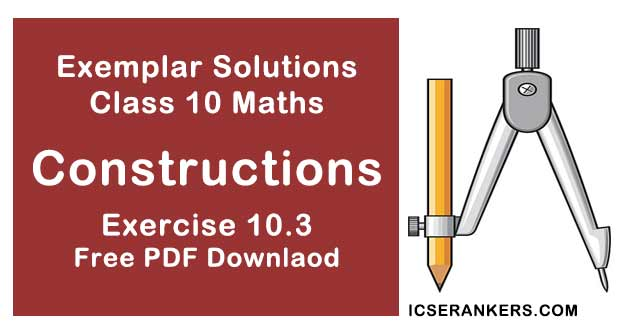Chapter Name NCERT Maths Exemplar Solutions for Chapter 10 Construction Exercise 10.3 Book Name NCERT Exemplar for Class 10 Maths Other Exercises Exercise 10.1Exercise 10.2Exercise 10.4 Related Study NCERT Solutions for Class 10 Maths

### Exercise 10.3 Solutions

1. Draw a line segment of length 7 cm. Find a point P on it which divides it in the ratio 3:5.

Solution

Steps of construction:

1. Draw a line segment AB = 7 cm.
2. Draw a ray AX, making an acute ∠BAX.
3. Along AX, mark 3 + 5 = 8 points A1 , A2 , A3 , A4 , A5 , A6 , A7 , A8
Such that AA1 = A1A2 = A2 A3 = A3A4 = A4A5 = A6A7 = A7A8
4. Join A8B.
5. From A3, draw A3P ॥ A8B meeting AB at P.
[by making an angle equal to ∠BA8A at A3]
So, P is the point on AB which divides it in the ratio 3 : 5.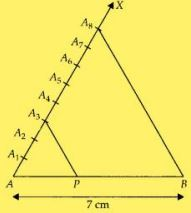Explanation :
Let
AA1 = A1A2 = A2 A3 = A3A4 ........... = A7A8 = x
In ∆ABA8 , we have
A3P || A8B
AP/PB = AA3/A3A8 = 3x/5x
Therefore, AP : PB = 3 : 5

2. Draw a right triangle ABC in which BC = 12 cm, AB = 5 cm and ∠B = 90°. Construct a triangle similar to it and of scale factor 2/3 . Is the new triangle also a right triangle?

Solution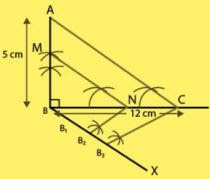Steps of construction:

1. Draw a line segment BC = 12 cm.
2. From B draw a line AB = 5 cm which makes right angle at B.
3. Join AC, ∆ABC is the given right triangle.
4. From B draw an acute ∠CBX downwards.
5. On ray BX, mark three points B1 , B2 and B3 , such that BB1 = B1B2 = B2B3 .
6. Join B3C.
7. From point B2 draw B2N ॥ B3C intersect BC at N.
8. From point N draw NM ॥ CA intersect BA at M. ∆MBN is the required triangle. ∆MBN is also a right angled triangle at B.

3. Draw a triangle ABC in which BC = 6 cm, CA = 5 cm and AB = 4 cm.
Construct a triangle similar to it and of scale factor 5/3 .

Solution

Steps of construction:

1. Draw a line segment BC = 6 cm.
2. Taking B and C as centers, draw two arcs of radii 4 cm and 5 cm intersecting each other at A.
3. Join BA and CA. ∆ABC is the required triangle.
4. From B, draw any ray BX downwards making at acute angle ∠CBX
5. Mark five points B1 , B2 , B3 , B4  and B5 on BX, such that BB1 = B1B2 = B2B3  = B3B4 = B4B5.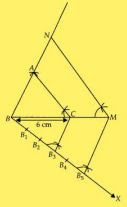6. Join B3C and from B5  draw B5M ॥ B3C intersecting the extended line segment BC at M.
7. From point M draw MN ॥ CA intersecting the extended line segment BA at N.

Therefore, ∆NBM is the required triangle whose sides is equal to 5/3 of the corresponding sides of the ∆ABC.

4. Construct a tangent to a circle of radius 4 cm from a point which is at a distance of 6 cm from its center.

Solution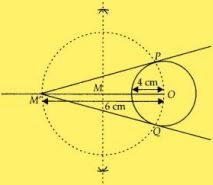We have, a point M’ is at a distance of 6 cm from the centre of a circle of radius 4 cm.
Steps of construction:

1. Draw a circle of radius 4 cm. Let the centre of this circle be O.
2. Join OM’ and bisect it. Let M be mid-point of OM’.
3. Taking M as centre and MO as radius draw a circle to intersect circle (0, 4) at two points, P and Q.
4. Join PM’ and QM’. PM’ and QM’ are the required tangents from M’ to circle C(0, 4).